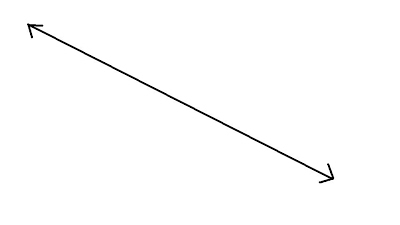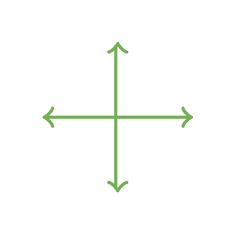100

12 x 5=

60

100What is this called?

A line

100

1/4 + 2/4=

3/4

100

Sum

100

What is Ms . Corey afraid of ?

Cats

200

9 x 9=

81

200

A right angle is at what degrees?

90 degrees

200

1/8 + 6/8=

7/8

200

Difference

200

When is the last day of summer learning academy ?

Tomorrow or July 29th

300

13 X 85=

1,105

300
What is the angle called that is less than 90 degree?

Acute

300

6/9 + 2/9=

8/9

300

Product or Multiple

300

Convert the following fraction into a decimal

3/4 is equal to

0.75

400

12 x 8 =

96
400What do you call this image?

perpendicular lines

400

3/7 + 4/7 =

7/7 or 1

400

Quotient

400

Convert the follow fraction into a decimal

6/24=

0.25

500

13 x 10=

130

500

What is the degree of a circle?

360

500

1/ 2 + 3/6=

6/6 or 1=

500

What is a line segment?

portion or part of a line with two end points

500

What day is the first day of school for the 2021-2022 school year ?

September 9th

Click to zoom